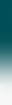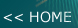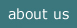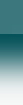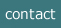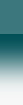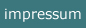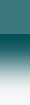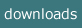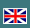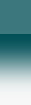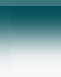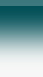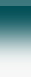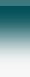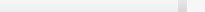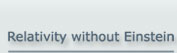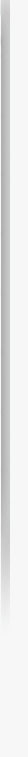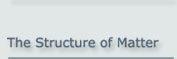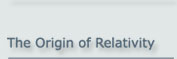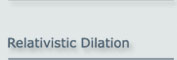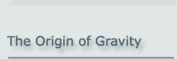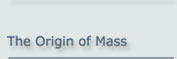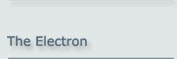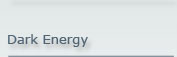Relativistic Contraction without Einstein!

What does it mean? - The 4-dimensional space-time is not necessary to explain
the effect of contraction!

The relativistic contraction, the so called 'Lorentz Contraction', is the consequence of the contraction of fields at motion.

The spatial extension of any object in the universe is caused by fields, in general by multi pole fields, which build a bond at a separation between those particles that constitute the object. This is true for the atoms in a molecule, for the elementary particles in the nucleus and for the basic particles (e.g. quarks) in an elementary particle.

The explanation of Einstein that the space itself contracts is in this view a kind of an overkill. It is unnecessary.

(Note: This page is also available as a Adobe Acrobat document.)

1. Relativistic Attenuation of a Field in Motion

According to the Theory of Relativity, for any kind of a field the field strength decreases - or in other words, the field contracts - as soon as the field is in motion. The term 'field in motion' will be used here for a field which is caused by moving charges.

In the view of today's particle physics the field force is the result of the interaction with exchange particles which are emitted by every charge and received by the other charges. The force acting on a particle and therefore the field strength at a certain position is the result of the density of the exchange particle stream at that point.

Using this fact, the relativistic change of a field can be deduced from the changes that are caused by the density change of the exchange particle as a result of motion. For this deduction it is assumed that the exchange particles are moving with the speed of light in relation to a fixed frame. So the following is compatible with the assumption of an ether as a general reference of motion, and it is in compliance with the so called 'Lorentzian Interpretation of the Theory of Relativity' .

The change of density of a stream of exchange particles which are radiated from a charge in motion is caused by two effects:

1. The path of an exchange particle which flies from the charge in the front to the charge in the rear is shortened. Correspondingly the opposite path from the charge in the rear to the charge in the front is extended.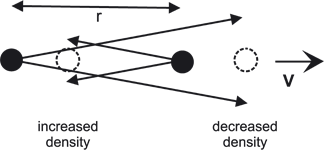2. Due to the aberration effect the density of the exchange particles, which is assumed to be isotropic at rest, will increase in the direction of motion and decrease in the adverse direction.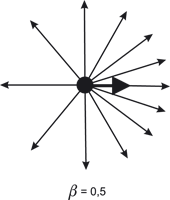We will now determine both effects quantitatively.

2. The path change for an exchange particle in motion

If both charges are moving at a speed of v, but the distance of both charges remains r, then the path length to be passed by the exchange particle from the rear particle to the one in front is extended to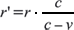.
This is caused by the fact that the speed of the exchange particle, which is c in relation to the system at rest, is
c - v
in relation to the motion of the charges.

Equivalently the path length to be passed by the exchange particle from the front particle to the one in the rear is reduced to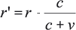.
This means that the density of the exchange particles arriving at the 'destination' charge will be increased or decreased respectively due to the effective change of the path length.

3. Density change of an exchange particle by aberration

It is supposed that the exchange particles emerging form a charge are equally (i.e. isotropicly) distributed into every spatial direction. However, if the emitting charge is moving, there will be an increase of density in the direction of motion and a decrease in the opposite direction as described by the aberration formula.

If an exchange particle is emitted in the direction α in relation to the x-axis, α can be described by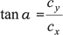where cx and cy are the x and y component respectively of the exchange particle's speed moving with the speed of light c.

For a charge moving at speed v, the angle α will, due to the geometry of the aberration process, change to α' so that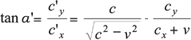.
From this it follows that: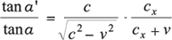.
On the other hand:This inserted yields:For the case considered here those directions of the exchange particles are essential, that are close either to the direction of motion or to the opposite direction:

1.α 0 degrees. Then cx c, tan αα, tan α'α', cos α ≈ 1 and therefore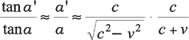2. α 180 degrees, i.e. v in the opposite direction and so with the sign inverted against cx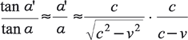.
In the first case the angle will be smaller, this means a higher density of the particle stream, which will be inversely proportional to the square of the angle. In latter case it means a corresponding dilution.

4. Combined effect of density change and aberration

The combination of the effects of the change of the path length and of the aberration is the product of both contributions. For the density change G of the rear particle onto the front particle this means: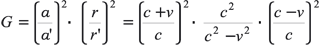.
where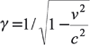.
For the influence of the front particle to the rear particle it means correspondingly:with the same result as above.

So the reduction of the field is the same in either direction, in the direction of the general motion and opposite to it. This reduction by γ2 will be compensated by a reduction of the distance by a factor of γ.

This is meant by the statement of the theory of relativity, that a field 'contracts' in motion.

Note: This understanding of contraction is used in the which explains the Special Relativity without the assumptions of Einstein about the four-dimensional space-time.

Bibliographical Reference

 F. Selleri et al., Die Einsteinsche und lorentzianische Interpretation der speziellen und allgemeinen Relativitätstheorie. Karlsbad:VRI 1998 (Germany).

The concept of the Basic Model of matter was initially presented at the Spring Conference of the German Physical Society (Deutsche Physikalische Gesellschaft) on 24 March 2000 in Dresden by Dr. Albrecht Giese.

Comments are welcome.

2011-12-20